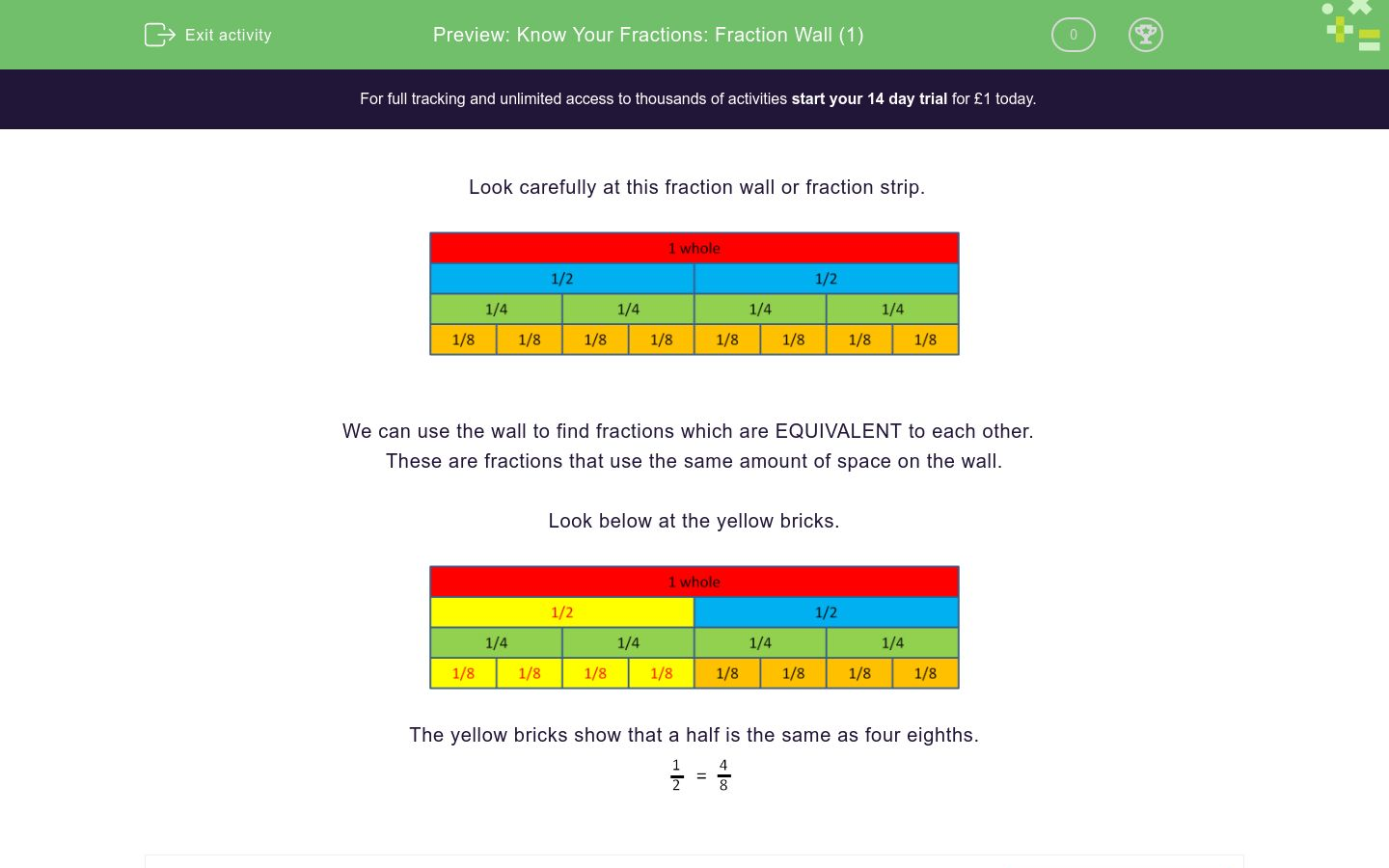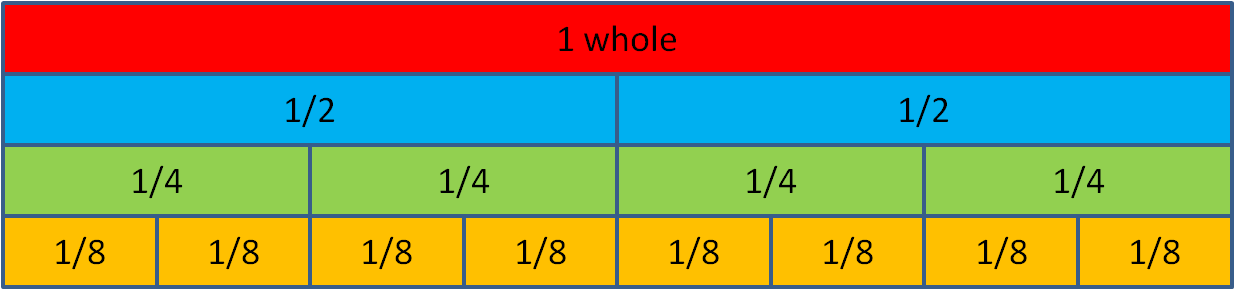# Know Your Fractions: Fraction Wall (1)

In this worksheet, students use a fraction wall to find equivalent fractions.Key stage:  KS 2

Curriculum topic:   Number: Fractions

Curriculum subtopic:   Use Fractions

Difficulty level:### QUESTION 1 of 10

Look carefully at this fraction wall or fraction strip.We can use the wall to find fractions which are EQUIVALENT to each other.

These are fractions that use the same amount of space on the wall.

Look below at the yellow bricks.The yellow bricks show that a half is the same as four eighths.Use the fraction wall to write down how many halves there are in a whole.Use the fraction wall to write down how many eighths there are in a whole.Use the fraction wall to write down how many quarters there are in a whole.Use the fraction wall to write down how many quarters there are in a half.Use the fraction wall to write down how many quarters there are in two halves.Use the fraction wall to write down how many eighths there are in a half.Use the fraction wall to write down how many eighths there are in two halves.Use the fraction wall to write down how many eighths there are in a quarter.Use the fraction wall to write down how many eighths there are in two quarters.Use the fraction wall to write down how many halves there are in two quarters.• Question 1

Use the fraction wall to write down how many halves there are in a whole.2
EDDIE SAYS
There are two halves in one whole.
• Question 2

Use the fraction wall to write down how many eighths there are in a whole.8
EDDIE SAYS
There are eight orange sections in one whole.
• Question 3

Use the fraction wall to write down how many quarters there are in a whole.4
EDDIE SAYS
There are four green sections (quarters) in one whole.
• Question 4

Use the fraction wall to write down how many quarters there are in a half.2
EDDIE SAYS
There are two green 1/4 sections under one blue 1/2 section, which means there are two quaters in a half.
• Question 5

Use the fraction wall to write down how many quarters there are in two halves.4
EDDIE SAYS
There are four quarters in two halves, which is the same as one whole.
• Question 6

Use the fraction wall to write down how many eighths there are in a half.4
EDDIE SAYS
There are four eighths in one half. You can see this in the fraction wall, as there are four orange sections under one big blue section.
• Question 7

Use the fraction wall to write down how many eighths there are in two halves.8
EDDIE SAYS
You can see that there are 8 orange sections under the 2 blue ones, so there are 8 eights in 2 halves.
• Question 8

Use the fraction wall to write down how many eighths there are in a quarter.2
EDDIE SAYS
There are two eights in a quarter. You can see this here as there are two orange sections under every green section.
• Question 9

Use the fraction wall to write down how many eighths there are in two quarters.4
EDDIE SAYS
Again, there are two orange sections under every green section, so there are 4 orange sections under 2 green sections. This means there are 4 eights in 2 quarters.
• Question 10

Use the fraction wall to write down how many halves there are in two quarters.1
EDDIE SAYS
You can see from the fraction wall that, above every two green sections, there is one blue section. This means that there is one half for every 2 quarters.
---- OR ----

Sign up for a £1 trial so you can track and measure your child's progress on this activity.

### What is EdPlace?

We're your National Curriculum aligned online education content provider helping each child succeed in English, maths and science from year 1 to GCSE. With an EdPlace account you’ll be able to track and measure progress, helping each child achieve their best. We build confidence and attainment by personalising each child’s learning at a level that suits them.

Get started